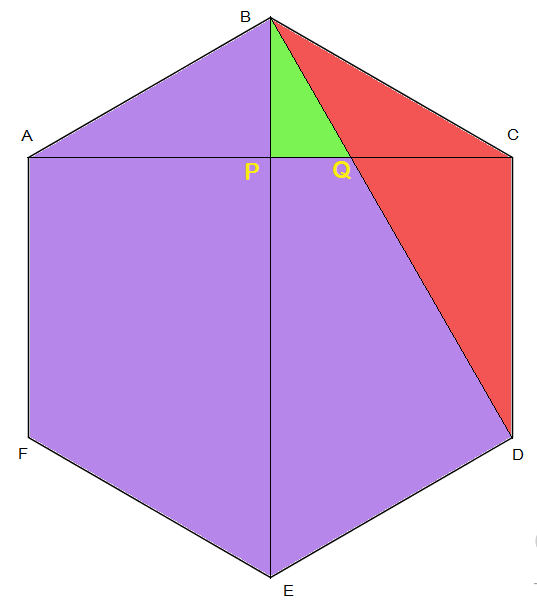# (Color+beauti)ful Hexagon!

Geometry Level 4As shown in the above figure, in a regular hexagon $ABCDEF$, the segments $BD, BE, CA$ are drawn.

$BD$ and $CA$ intersect at point $Q$.

$BE$ and $CA$ intersect at point $P$.

$A(\triangle BPQ)$ is colored $\color{#20A900}{\text{green}}$

$A(\triangle BCD)$ is colored $\color{#D61F06}{\text{red}}$

Rest of the area is colored $\color{#69047E}{\text{purple}}$

If the ratio of areas of colors

$\color{#69047E}{\text{purple}}:\color{#20A900}{\text{green}}:\color{#D61F06}{\text{red}}=\color{#69047E}{a}:\color{#20A900}{b}:\color{#D61F06}{c}$

such that $a,b,c \in \mathbb{N}$ , $\text{gcd}(a,b,c)=1$.

Find $a+b+c$

×